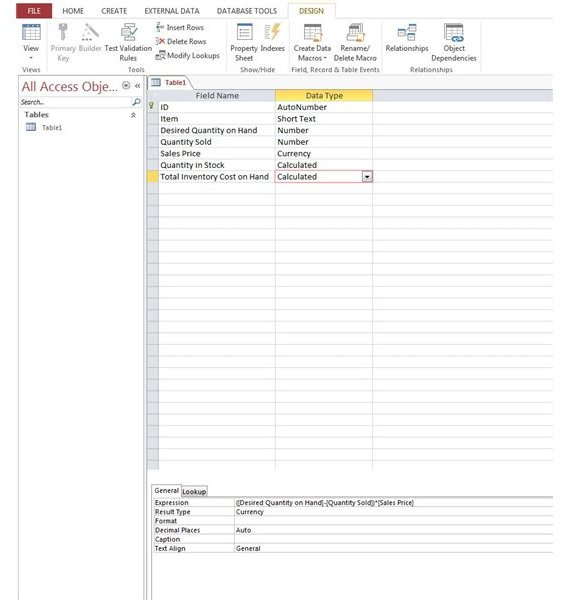# Defining and Using Calculated Fields in Access 2013: Tutorial With Examples

Page content

## Setting the Stage

So… what is a calculated field? In short, these fields are created by performing a calculation on one or more existing fields. A calculation can be as simple as finding an average of a set of fields or as complex as a nested expression containing multiple functions.

For the purposes of this article, I’ll walk you through creating a few calculated fields using a sample inventory database. One of our columns will contain the number of items we want to keep in stock while a second column will contain the number of items sold. We want to create a calculated column to determine the current number of items in stock. Then we’ll take it another step further and create a calculated column to show total revenue of items sold using a nested expression.

## Getting Started

First let’s set up our sample database.

1. Open Access and create a Blank Desktop database.

2. Switch to Design View.

3. Create the following fields: Item, Desired Quantity On Hand, Quantity Sold and Sales Price. Item will be a Short Text data type while the Desired Quantity and Quantity Sold will be Number Data Type. Sales Price will use the Currency Data Type (Figure 1).

4. Save the table and switch back to Datasheet view.

5. Enter in some sample data (Figure 2).

## Creating the Calculated Fields

Follow these steps to add our new calculated fields. We’ll create our first calculated column which will subtract Desired Quantity from Quantity Sold.

1. Click the Design View.

2. Create a new field called “Quantity in Stock” and select the Calculated Data Type.

3. The expression builder will open. We can select fields by using the field name enclosed by squares brackets [] along with the function we want to perform. You can also double click the field name in the “Expression Categories” section. In the expression builder type the following: [Desired Quantity on Hand] – [Quantity Sold] and click OK (Figure 3).

4. Click back to Datasheet view to see the results of our calculated field (Figure 4).5. Next, click back to Design view.

6. Create a new field called “Total Inventory Cost on Hand” with a Calculated Data Type.

7. In the Expression Builder we’ll use a nested expression. Although we could have just used our newly created “Quantity in stock” field for this exercise, I figured it’d be nice to show a nested example. To create a nested expression, we’ll put parentheses around our first calculation and then perform our second operation. The expression will look like this:

([Desired Quantity on Hand]-[Quantity Sold])*[Sales Price]

8. As you can imagine, Access will first calculate Desired Quantity minus Quantity sold to give us total number of units on hand. We then multiply this number by the Sales price to get our inventory cost of on hand items (Figure 5).

9. Save the table and click back to the datasheet view to see our new column (Figure 6).

Keep in mind Access has a ton of different functions you can use on your fields to come up with some pretty complex calculated columns. Just remember to use parentheses to let Access know which operations need to be calculated first. You can use multiple sets of parentheses to build multi-leveled expressions.

That’s the basics of using calculated columns! Have any tips of your own to share? Leave us a note in the comments.